Courses

NCERT Solution (EX- 2.5) - Chapter 2: Linear Equations in one variable, Maths, Class 8 Notes - Class 8

Class 8: NCERT Solution (EX- 2.5) - Chapter 2: Linear Equations in one variable, Maths, Class 8 Notes - Class 8

The document NCERT Solution (EX- 2.5) - Chapter 2: Linear Equations in one variable, Maths, Class 8 Notes - Class 8 is a part of Class 8 category.
All you need of Class 8 at this link: Class 8

Linear Equations in one variable

Exercise 2.5

Question 1:
Solve the following linear equation: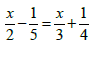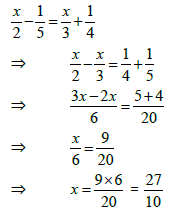To check: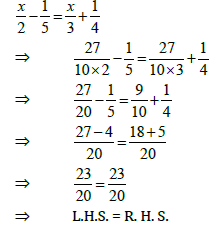Therefore, it is correct.

Question 2:
Solve the following linear equation: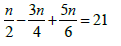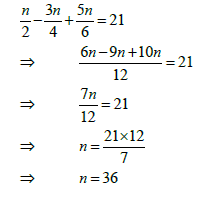To check: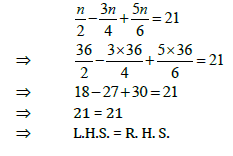Therefore, it is correct.

Question 3:
Solve the following linear equation: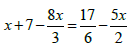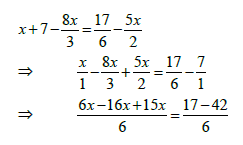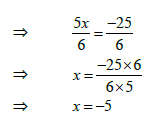To check: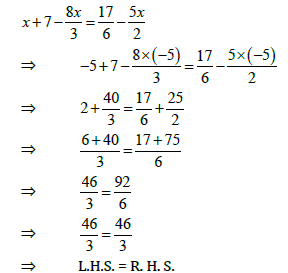Therefore, it is correct.

Question 4:
Solve the following linear equation: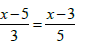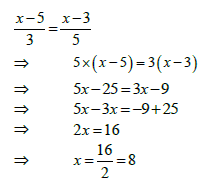To check: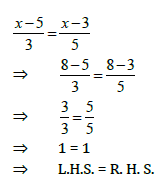Therefore, it is correct.

Question 5:
Solve the following linear equation :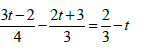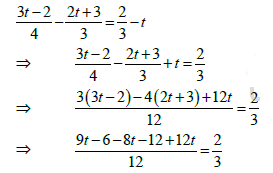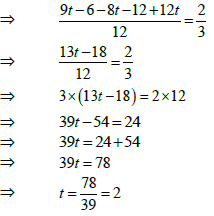To check: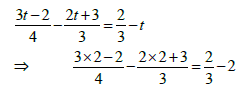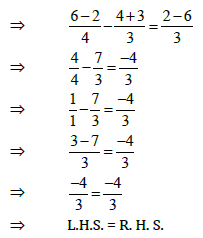Therefore, it is correct.

Question 6:
Solve the following linear equation: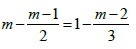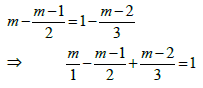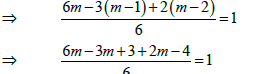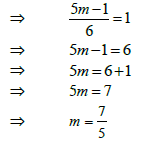To check: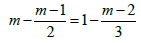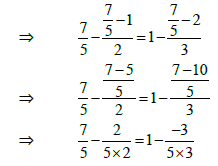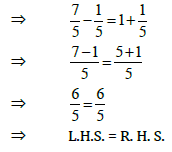Therefore, it is correct.

Question 7:
Simplify and solve the following linear equation: 3(t-3) = 5(2t+1)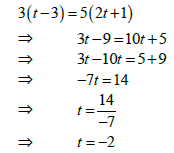To check: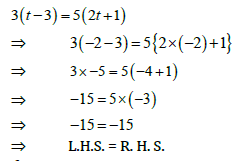Therefore, it is correct.

Question 8:
Simplify and solve the following linear equation: 15(y-4)-2(y-9)+5(y+6) =0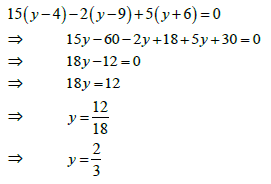To check: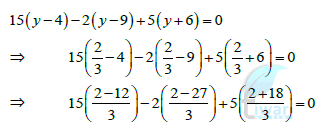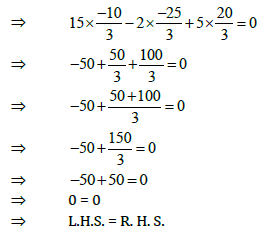Therefore, it is correct.

Question 9:
Simplify and solve the following linear equation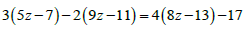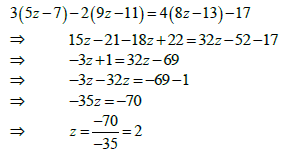To check: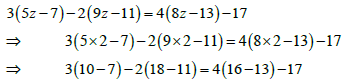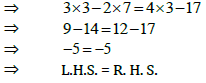Therefore, it is correct.

Question 10:
Simplify and solve the following linear equation: 0.25(f-3) =0.05(10f-9)

0.25(f-3) =0.05(10f-9)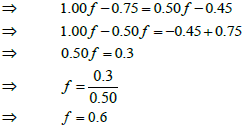To check:
0.25(4f-3)= 0.05(10f-9)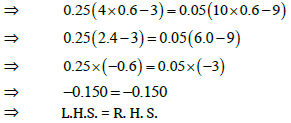Therefore, it is correct.

The document NCERT Solution (EX- 2.5) - Chapter 2: Linear Equations in one variable, Maths, Class 8 Notes - Class 8 is a part of Class 8 category.
All you need of Class 8 at this link: Class 8Use Code STAYHOME200 and get INR 200 additional OFF Use Coupon Code

Top Courses for Class 8Top Courses for Class 8Track your progress, build streaks, highlight & save important lessons and more!

,

,

,

,

,

,

,

,

,

,

,

,

,

,

,

,

,

,

,

,

,

,

,

,

,

,

,

;